Courses

# Quant Mock Test - 17

## 15 Questions MCQ Test Mock Test Series for CLAT 2020 | Quant Mock Test - 17

Description
This mock test of Quant Mock Test - 17 for CLAT helps you for every CLAT entrance exam. This contains 15 Multiple Choice Questions for CLAT Quant Mock Test - 17 (mcq) to study with solutions a complete question bank. The solved questions answers in this Quant Mock Test - 17 quiz give you a good mix of easy questions and tough questions. CLAT students definitely take this Quant Mock Test - 17 exercise for a better result in the exam. You can find other Quant Mock Test - 17 extra questions, long questions & short questions for CLAT on EduRev as well by searching above.
QUESTION: 1

### Directions: Study the following graph carefully to answer the questions that follow.  Average monthly expenditure of an organisation under various Heads Total Expenditure Rs. 18, 50, 000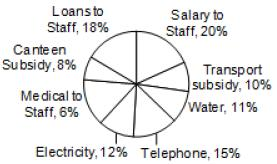Q. What is the amount spent on transport subsidy and canteen subsidy together?

Solution:

Amount spent on transport subsidy and canteen subsidy together = (10% + 8%) of 1850000
= 18% of 1850000 = 18/100 x 1850000 = 333000

QUESTION: 2

### Directions: Study the following graph carefully to answer the questions that follow.  Average monthly expenditure of an organisation under various Heads Total Expenditure Rs. 18, 50, 000Q. What is the difference between the expenditure on salary to staff and that on loans to staff?

Solution:

difference between the expenditure as salary to staff and that on loans to staff = (20% - 18%) of 1850000 = 20% of 1850000 = 2/100 x 1850000 = 2 x 185000 = Rs. 37000

QUESTION: 3

### Directions: Study the following graph carefully to answer the questions that follow.  Average monthly expenditure of an organisation under various Heads Total Expenditure Rs. 18, 50, 000Q. The amount spent on medical to staff is what percent of the amount spent on salary?

Solution:

Percentage = 6% / 20% x 100 = 30%

QUESTION: 4

Directions: Study the following graph carefully to answer the questions that follow.
Average monthly expenditure of an organisation under various Heads Total Expenditure Rs. 18, 50, 000Q. What is the amount spent on telephone?

Solution:

Amount spent on telephone = 15% of 1850000
= 15/100 x 1850000 = Rs 277500

QUESTION: 5

Directions: Study the following graph carefully to answer the questions that follow.
Average monthly expenditure of an organisation under various Heads Total Expenditure Rs. 18, 50, 000Q. What is the total expenditure on electricity and water together?

Solution:

Total expenditure on electricity and water together = (12% + 11%) of 1850000 = 23% of 1850000
= 23/100 x 185000 = 23 x Rs 18500 = Rs. 425500

QUESTION: 6

Directions: Show below is the multiple bar diagram depicting the changes in the student’s strength of a college in four faculties from 2000-01 to 2002-03.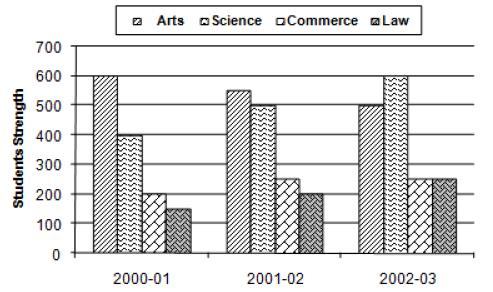Study the above multiple bar chart and mark a tick against the correct answer in each of the following questions.

Q. The percentage of students in science faculty in 2000-01 was:

Solution:

Total number of students in 2000-01 = (600 + 400 + 200 + 150) = 1350.
Number of science students in 2000-01 was 400.
Percentage of science students in 2000-01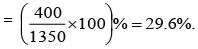QUESTION: 7

Directions: Show below is the multiple bar diagram depicting the changes in the student’s strength of a college in four faculties from 2000-01 to 2002-03.Study the above multiple bar chart and mark a tick against the correct answer in each of the following questions.

Q. The percentage of students in law faculty in 2002-03 was:

Solution:

Total number of students in 2002-03 = (500 + 600 + 250 + 250) = 1600.
Number of law students in 2002-03 is 250.
Percentage of law students in 2002-03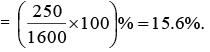QUESTION: 8

Directions: Show below is the multiple bar diagram depicting the changes in the student’s strength of a college in four faculties from 2000-01 to 2002-03.Study the above multiple bar chart and mark a tick against the correct answer in each of the following questions.

Q. How many times the total strength was of the strength of commerce students in 2001-02?

Solution:

Total strength in 2001–02 = (550 + 500 + 250 + 200) = 1500.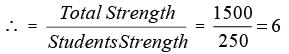QUESTION: 9

Directions: Show below is the multiple bar diagram depicting the changes in the student’s strength of a college in four faculties from 2000-01 to 2002-03.Study the above multiple bar chart and mark a tick against the correct answer in each of the following questions.

Q. During which year the strength of arts faculty was minimum?

Solution:

A slight look indicates that the strength in arts faculty in 2000-01, 2001-02 & 2002-03 was 600, 550 and 500 respectively.
So, it was minimum in 2002-03.

QUESTION: 10

Directions: Show below is the multiple bar diagram depicting the changes in the student’s strength of a college in four faculties from 2000-01 to 2002-03.Study the above multiple bar chart and mark a tick against the correct answer in each of the following questions.

Q. How much per cent was the increase in science students in 2002-03 over 2000-01?

Solution:

Number of science students in 2000-01 was 400.
Number of science students in 2002-03 was 600.
Percentage increase =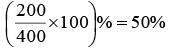QUESTION: 11

Directions: In the following graph, students passing in five years 2001 to 2005 from two schools A and B are given. On the basis of this graph, answer the following questions.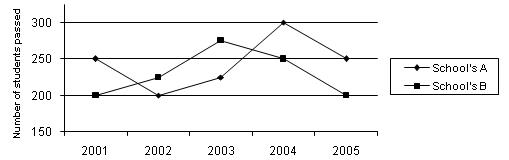Q. What is the total no. of students passed from both schools during 2002 to 2004?

Solution:

Total passed students from school A = 200 + 225 + 300 = 725
Total passed students from school B = 225 + 275 + 250 = 750
∴ Total = 1475

QUESTION: 12

Directions: In the following graph, students passing in five years 2001 to 2005 from two schools A and B are given. On the basis of this graph, answer the following questions.Q. The ratio of students passed from school A and those from school B in the years 2003 and 2005 together is

Solution:

1 : 1 A’s students : B’s students = (225 + 250) : (275 + 200)
= 475 : 475 ⇒ 1 : 1

QUESTION: 13

Directions: In the following graph, students passing in five years 2001 to 2005 from two schools A and B are given. On the basis of this graph, answer the following questions.Q. The average of students passed from school B during 2002 to 2004 is

Solution: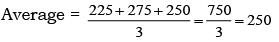QUESTION: 14

Directions: In the following graph, students passing in five years 2001 to 2005 from two schools A and B are given. On the basis of this graph, answer the following questions.Q. The total no. of students passed from both the schools during the whole period of 2001to 2005 is

Solution:

Total A = 250 + 200 + 225 +300 + 250 = 1225
Total B = 200 + 225 + 275 +250 + 200 = 1150
∴ Total = 1225 + 1150 = 2375

QUESTION: 15

Directions: In the following graph, students passing in five years 2001 to 2005 from two schools A and B are given. On the basis of this graph, answer the following questions.Q. Find the ratio of the maximum number of students passed in any year during the whole period 2001 to 2005 form school ‘A’ to that from school ‘B’.

Solution:

Max students passed from A is 300 in 2004.
Max students passed from B is 275 in 2003
∴Ratio is 300 / 275 ⇒ 12 : 11.